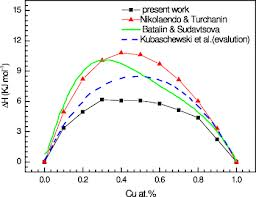Physics
Science

# Temperature Dependence of the Self-Diffusion Coefficient of liquid Fe.ABSTRACT

Temperature dependence of the self-diffusion coefficient D of liquid Fe has been investigated in this thesis work. Three different theories namely: Linear Trajectory, Small-step diffusion and Hard Sphere theory were used for this purpose. The ingredients for calculating the self-diffusion coefficient for the three methods mentioned above are the pair potential  and pair correlation function g(r). For calculating the self-diffusion coefficient using linear trajectory and small step diffusion theory the pair potential was obtained by Brettonet-Silbert (BS) pseudo-potential method while pair correlation function g(r) was obtained using VMHNC theory. On the other hand for the hard sphere theory  and g(r) was obtained from BS model and LWCA theory respectively. A comparison of the calculated diffusion coefficient D along with the data obtained by protopapas et al. shows that the self-diffusion coefficient increases with respect to increasing temperature. Also agreement among data obtained by small step and hard sphere theory and those of protopapas et al. is much better in comparison to the data obtained by linear trajectory theory.

Introduction

The temperature dependence of diffusion coefficient for liquid metals is an interesting phenomenon now-a-days. Both surfaces and liquid metals have some cohesively undetermined properties which attract the scientist very much. For that reason we have motivated our attention on this topic.

The surface of a substance is an atomic layer at which this substance is in contact with the surrounding world, in the ideal case, the vacuum. At a fundamental level, surfaces are of great interest because they represent a rather special kind of defect. The introduction of surface breaks the periodicity in one direction and can lead to structural changes as well as the introduction of localized electronic and vibrational states. A surface, in the mathematical sense, cannot have a structure, so by surface structure we mean the structure of solid in the vicinity of the surface. For this reason, it is useful to define a region of the solid in the vicinity of the mathematical surface as the selvedge (by analogy with the edge of a piece of a cloth) Many properties of solids are intimately related to the special symmetry properties of bulk crystalline materials.  While a solid surface is intrinsically an imperfection of a crystalline solid, destroying the three dimensional periodicity (parallel to the surface) and this periodicity is an important factor in determining some of the properties of the surface. In particular, it plays a dominant role in allowing electron, X-ray and atom diffraction techniques to provide information on the structure of the surface, as well as strongly influencing the electronic properties of the surface.

Liquid metals are interesting in a sense that they are composed of a collection of the atoms of electropositive elements, and they are distinguished from solid by their atomic arrangement which is irregular.

But we are now dealing with a problem of determining diffusion coefficient of transition metals at different temperatures. The structures of liquid surfaces have not yet been conclusively determined but study is ongoing. To understand the surface properties of liquid metal it is necessary to investigate carefully the electronic contribution to the surface energy. The work of Lang and Khon (1970) appears to give an important basis on this problem.

The diffusion coefficient of a simple liquid metal is a quantity of continued research interest. Previous study of diffusion coefficient relies on finding a reference system which is characterized by one or more parameters chosen to model the real system. The Linearized version of the Weeks-Chandler-Anderson (LWCA) thermodynamic perturbation scheme is one such earlier theory that belongs to this type. Hard sphere model has also been used to calculate entropy. In this report, we have extended this theory for surface with a more theoretical approach proposed by Protopapas et al. The surface tension at the surface differs from that of bulk because the fewer neighbors of the surface cause it to have a very different and anisotropic chemical environment compared to the bulk. When an atom moves from the bulk to the surface, the area of the surface increases. Therefore we have to add an additional term which is surface tension times increasing area. But one important thing is that the specific surface energy is independent on the surface. Thus from thermodynamics it can be shown that when it varies with temperature or alternatively the temperature dependence of surface tension leads to the surface entropy. For the calculation of surface entropy of liquid metal, T. Itami and M. Shimoji  derived an expression where they kept packing fraction (